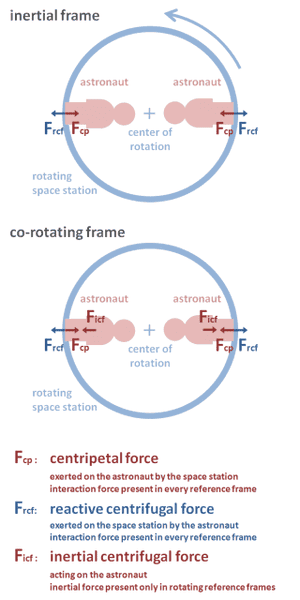# Centripetal and Centrifugal force

I just read that Centrifugal Force is a false force. It does not exist. Inertia (which is not a force) is mistakenly called Centrifugal force. Now, here is my question

According to Newton's 3rd law of motion, forces always exist in pairs; action and reaction. Centripetal force which is the force acting on the body pushing it towards the center must have a pair that is the force acting on the object that is exerting the centripetal force. For example: a yo-yo twirled around a finger is pulled along the string by the finger towards the finger - centripetal force. The force with which the yo-yo pulls on the finger along the string What is that force called then? This has nothing to do with the inertia of the yo-yo which will make it fly along a straight line as soon as the centripetal force is moved.

A.T.
I just read that Centrifugal Force is a false force. It does not exist.
It is an inertial force, not an interaction force. In a rotating frame it "exists" in the same sense as interaction forces do:
http://en.wikipedia.org/wiki/Centrifugal_force_(rotating_reference_frame)
Inertia (which is not a force) is mistakenly called Centrifugal force.
It is not a "mistake" but a practical mathematical concept:
http://en.wikipedia.org/wiki/Fictitious_force

According to Newton's 3rd law of motion, forces always exist in pairs; action and reaction.
Note that this law applies only to interaction forces, not inertial forces. But you are correct that the interaction centripetal force must have a equal but opposite force, according to Newtons 3rd.

Centripetal force which is the force acting on the body pushing it towards the center must have a pair that is the force acting on the object that is exerting the centripetal force. For example: a yo-yo twirled around a finger is pulled along the string by the finger towards the finger - centripetal force. The force with which the yo-yo pulls on the finger along the string What is that force called then?
Some call it "reactive centrifugal force":
http://en.wikipedia.org/wiki/Reactive_centrifugal_force

Note that contrary to the "inertial centrifugal force" in a rotating ref. frame this "reactive centrifugal force" is an interaction force that exists in all ref. frames. See the table in this section for a comparison of the two:
http://en.wikipedia.org/wiki/Reactive_centrifugal_force#Relation_to_inertial_centrifugal_force

Here another example to explain all three forces, a rotating space station with two astronauts:Last edited:
tiny-tim
Homework Helper
hi kghosh!I just read that Centrifugal Force is a false force. It does not exist. Inertia (which is not a force) is mistakenly called Centrifugal force.

there is no centrifugal force for an inertial observer

there is centrifugal force for an observer in a rotating frame

centrifugal force is a fictititious (or inertial) force … some observers create it for their conveniencea yo-yo twirled around a finger is pulled along the string by the finger towards the finger - centripetal force. The force with which the yo-yo pulls on the finger along the string What is that force called then?

just a reaction force